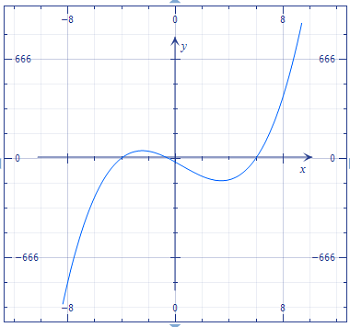# {eq}f(x) = 2x^3-3x^2-50x-26{/eq}. Find all complex zeros. (a) {eq}x ={/eq} (b) {eq}f(x) ={/eq}

## Question:

{eq}f(x) = 2x^3-3x^2-50x-26{/eq}.

Find all complex zeros. (a) {eq}x ={/eq} (b) {eq}f(x) ={/eq}

## Cubic Functions:

Recall the the fundamental theorem of algebra ensures us that all cubic functions have exactly three roots, where we either have three real roots, or one real and two complex roots (where the complex roots are complex conjugates of one another). Since we have the technology, the best way to get a leg up on these functions is to take a look at what they look like.

This whole "(a) x= (b) f(x) =" business is silly. We know we are looking for the zeroes, so we want to know the {eq}x {/eq} values when {eq}f (x) = 0 {/eq}. So obviously {eq}f (x) = 0 {/eq}. If the point was to be needlessly confusing, then job well done.

Our function is

{eq}\begin{align*} y &= 2x^{3}-3x^{2}-50x-26 \end{align*} {/eq}

Let's take a look at it.Ugh, this is messed up; we are being hoodwinked. Clearly, the graph passes through {eq}y = 0 {/eq} at three real points (we use our trace feature to see that they are approximately -3.97, -0.54, and 6.02). Thus there are no complex zeroes.